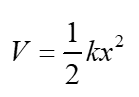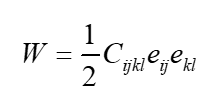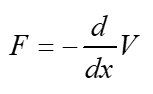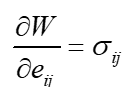# Strain Energy Functions and Springs

• Trying2Learn

#### Trying2Learn

TL;DR Summary
Strain energy and elastic constants: why is the strain energy formed this way
(If this is in the wrong forum, please move it)

Here is the potential energy of a springHere is the strain energy function in elasticityThe look alike -- I like that.

If we want the force in the spring, we take the derivative of V with respect to the displacement and make the result NEGATIVEHowever, we note the following property of the strain energy function (from Fung's Solid Mechancis, page 128)So, real stupid question: what happened to the negative?

I mean, I am trying to work my way through how elasticity, strain energy, etc. lead to the number of constants relating stress and strain. I will work this through on my own. I am trying to put all of this in some sort of contextual balance.

I can intuit the need for a strain energy function without the negative. But part of me wants to see the negative to make it harmonious with a spring.

Can someone get me out of this?

Try replacing the spring with a 1d bar. Upon extension, the bar experiences a tensile stress which produces a negative force on a point mass at the end of the bar/string.

The first equation looks at the force on a point mass. The force required to stretch the spring the relevant distance is the negative of this force.

Last edited:
•Trying2Learn
Ah, of course. Thank you!